# JP5141780B2 - Rotation angle sensor - Google Patents

## Info

Publication number
JP5141780B2
JP5141780B2 JP2011003552A JP2011003552A JP5141780B2 JP 5141780 B2 JP5141780 B2 JP 5141780B2 JP 2011003552 A JP2011003552 A JP 2011003552A JP 2011003552 A JP2011003552 A JP 2011003552A JP 5141780 B2 JP5141780 B2 JP 5141780B2
Authority
JP
Japan
Prior art keywords
magnet
magnetic field
magnetic sensor
rotation axis
ring
Prior art date
Legal status (The legal status is an assumption and is not a legal conclusion. Google has not performed a legal analysis and makes no representation as to the accuracy of the status listed.)
Active
Application number
JP2011003552A
Other languages
Japanese (ja)
Other versions
JP2012145425A (en
Inventor

Original Assignee
Ｔｄｋ株式会社
Priority date (The priority date is an assumption and is not a legal conclusion. Google has not performed a legal analysis and makes no representation as to the accuracy of the date listed.)
Filing date
Publication date
Application filed by Ｔｄｋ株式会社 filed Critical Ｔｄｋ株式会社
Priority to JP2011003552A priority Critical patent/JP5141780B2/en
Publication of JP2012145425A publication Critical patent/JP2012145425A/en
Application granted granted Critical
Publication of JP5141780B2 publication Critical patent/JP5141780B2/en
Active legal-status Critical Current
Anticipated expiration legal-status Critical

• 230000005291 magnetic Effects 0.000 claims abstract description 337
• 230000005415 magnetization Effects 0.000 claims abstract description 44
• 230000000694 effects Effects 0.000 claims description 12
• 238000000034 methods Methods 0.000 description 3
• 238000006243 chemical reactions Methods 0.000 description 2
• 238000004364 calculation methods Methods 0.000 description 1
• 238000005516 engineering processes Methods 0.000 description 1
• 238000004519 manufacturing process Methods 0.000 description 1

## Images

•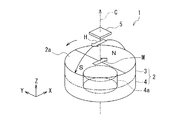•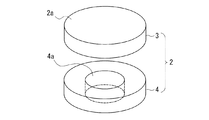••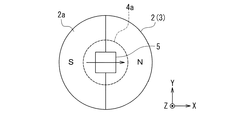•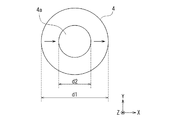•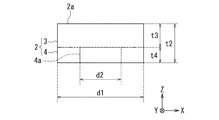•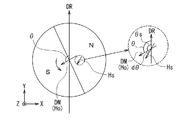•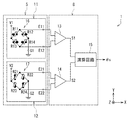•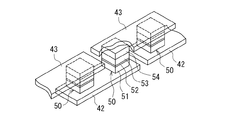•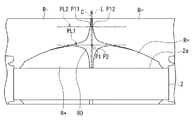•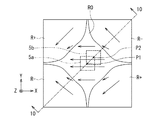•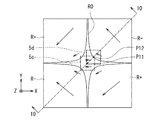•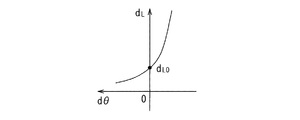•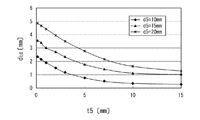•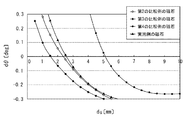•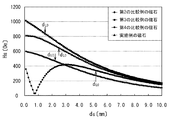•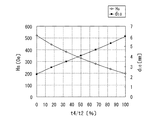•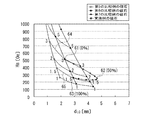•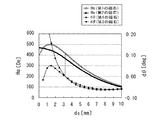•••••••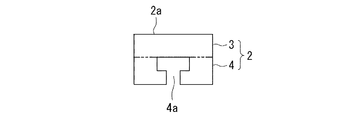•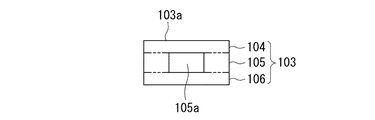## Classifications

• GPHYSICS
• G01MEASURING; TESTING
• G01RMEASURING ELECTRIC VARIABLES; MEASURING MAGNETIC VARIABLES
• G01R33/00Arrangements or instruments for measuring magnetic variables
• G01R33/02Measuring direction or magnitude of magnetic fields or magnetic flux
• G01R33/06Measuring direction or magnitude of magnetic fields or magnetic flux using galvano-magnetic devices, e.g. Hall effect devices; using magneto-resistive devices
• G01R33/09Magnetoresistive devices
• G01R33/091Constructional adaptation of the sensor to specific applications
• GPHYSICS
• G01MEASURING; TESTING
• G01DMEASURING NOT SPECIALLY ADAPTED FOR A SPECIFIC VARIABLE; ARRANGEMENTS FOR MEASURING TWO OR MORE VARIABLES NOT COVERED IN A SINGLE OTHER SUBCLASS; TARIFF METERING APPARATUS; MEASURING OR TESTING NOT OTHERWISE PROVIDED FOR
• G01D5/00Mechanical means for transferring the output of a sensing member; Means for converting the output of a sensing member to another variable where the form or nature of the sensing member does not constrain the means for converting; Transducers not specially adapted for a specific variable
• G01D5/12Mechanical means for transferring the output of a sensing member; Means for converting the output of a sensing member to another variable where the form or nature of the sensing member does not constrain the means for converting; Transducers not specially adapted for a specific variable using electric or magnetic means
• G01D5/14Mechanical means for transferring the output of a sensing member; Means for converting the output of a sensing member to another variable where the form or nature of the sensing member does not constrain the means for converting; Transducers not specially adapted for a specific variable using electric or magnetic means influencing the magnitude of a current or voltage
• G01D5/142Mechanical means for transferring the output of a sensing member; Means for converting the output of a sensing member to another variable where the form or nature of the sensing member does not constrain the means for converting; Transducers not specially adapted for a specific variable using electric or magnetic means influencing the magnitude of a current or voltage using Hall-effect devices
• G01D5/145Mechanical means for transferring the output of a sensing member; Means for converting the output of a sensing member to another variable where the form or nature of the sensing member does not constrain the means for converting; Transducers not specially adapted for a specific variable using electric or magnetic means influencing the magnitude of a current or voltage using Hall-effect devices influenced by the relative movement between the Hall device and magnetic fields
• GPHYSICS
• G01MEASURING; TESTING
• G01RMEASURING ELECTRIC VARIABLES; MEASURING MAGNETIC VARIABLES
• G01R33/00Arrangements or instruments for measuring magnetic variables
• G01R33/0011Arrangements or instruments for measuring magnetic variables comprising means, e.g. flux concentrators, flux guides, for guiding or concentrating the magnetic flux, e.g. to the magnetic sensor

## Abstract

A rotation angle sensor includes a magnet that rotates about a rotation axis. The magnet has an end face perpendicular to the rotation axis and has a magnetization in a direction perpendicular to the rotation axis. The rotation angle sensor further includes a magnetic sensor that faces the end face of the magnet and detects a magnetic field produced by the magnet. The magnet includes a plate-shaped portion including the end face, and a ring-shaped portion that is located on a side of the plate-shaped portion farther from the end face and coupled to the plate-shaped portion. The plate-shaped portion does not include any hollow through which the rotation axis passes, whereas the ring-shaped portion includes a hollow through which the rotation axis passes.

## Description

The present invention relates to a rotation angle sensor that includes a rotating magnet and a magnetic sensor that detects a magnetic field generated by the magnet, and detects a rotation angle of the magnet.

In recent years, magnetic rotation angle sensors have been widely used to detect the rotation position of an object in various applications such as detection of the rotation position of a steering wheel of an automobile. As a magnetic rotation angle sensor, for example, as described in Patent Documents 1 and 2, a rotating magnet and a magnetic sensor for detecting a magnetic field by the magnet are provided, and the magnet is based on the detection output of the magnetic sensor. A device that detects the rotation angle of the lens is known.

In a rotation angle sensor including a magnet and a magnetic sensor, for example, the magnet has an end surface perpendicular to the rotation axis and magnetization in a direction perpendicular to the rotation axis, and the magnetic sensor has a predetermined value relative to the end surface of the magnet. Are arranged so as to face each other with a gap therebetween. The performance required for the rotation angle sensor having such a configuration is that the intensity of the magnetic field generated by the magnet and applied to the magnetic sensor is large, and the detection is based on the actual rotation angle of the magnet and the detection output of the magnetic sensor. The difference (hereinafter referred to as an angle error) with respect to the rotation angle of the magnet (hereinafter referred to as a detection angle) is small.

In addition to the magnetic field generated by the magnet, other magnetic fields such as a leakage magnetic field from the motor and geomagnetism may be applied to the magnetic sensor. The strength of the magnetic field generated by the magnet and applied to the magnetic sensor is necessary to relatively reduce the influence of the magnetic field applied to the magnetic sensor other than the magnetic field generated by the magnet. . Increasing the strength of the magnetic field generated by the magnet and applied to the magnetic sensor is realized, for example, by forming the magnet with a magnetic material having a large residual magnetic flux density or increasing the size of the magnet.

On the other hand, one of the causes for causing an angle error is a shift in the positional relationship between the magnet and the magnetic sensor. The positional relationship between the magnet and the magnetic sensor may slightly deviate from the desired positional relationship when the rotational angle sensor is manufactured or when the rotational angle sensor is used. Here, it is assumed that the direction of the magnetic field by the magnet is parallel to the direction of magnetization of the magnet at a position where the rotation axes intersect on a virtual plane parallel to the end face of the magnet (hereinafter referred to as the center position). Then, on the virtual plane, the position of the magnetic sensor such that the rotation axis passes through the center of the magnetic sensor is set as a desired position of the magnetic sensor. On the virtual plane, at a position away from the center position, the direction of the magnetic field by the magnet may be different from the direction of the magnetic field by the magnet at the center position. Therefore, when the position of the magnetic sensor deviates from a desired position on the virtual plane, the direction of the magnetic field detected by the magnetic sensor is the magnetic field detected by the magnetic sensor when placed at the desired position. There may be cases where the direction is different. In this way, an angle error can occur due to a shift in the positional relationship between the magnet and the magnetic sensor. The rotation angle sensor is required to have a small angle error even when the positional relationship between the magnet and the magnetic sensor is shifted.

In Patent Document 1, in a rotation angle sensor having a disk magnet and a magnetoelectric conversion element, the magnetoelectric conversion element is arranged at a position where the angle error due to the rotational axis deviation of the disk magnet is reduced, thereby reducing the angle error. The technology to suppress is described.

Patent Document 2 describes a technique for reducing the angle error by devising the shape of the magnet and the arrangement of the magnetic detection units. In this technique, the magnet has a vicinity of the rotation center axis, a first outer periphery outside the vicinity, and a second outer periphery outside the first outer periphery. The thickness of the vicinity in the direction parallel to the rotation center axis is thicker than the thickness of the first outer periphery and the second outer periphery in the direction parallel to the rotation center axis.

JP 2007-93280 A JP 2010-66196 A

As described above, in the rotation angle sensor including the rotating magnet and the magnetic sensor, the strength of the magnetic field generated by the magnet and applied to the magnetic sensor is large, and the positional relationship between the magnet and the magnetic sensor is shifted. The required angle error is required to be small. In order to satisfy these requirements, it is conceivable to increase the intensity of the magnetic field generated by the magnet and to arrange the magnetic sensor at a position where the angular error due to the positional relationship between the magnet and the magnetic sensor is reduced. .

The inventors of the present application have shown by simulation that a region in which the direction of the magnetic field in the region is substantially the same as the direction of the magnetic field at a desired position (hereinafter referred to as a parallel magnetic field region). And the area of the parallel magnetic field region on the virtual plane parallel to the end surface of the magnet was confirmed to change depending on the distance between the end surface of the magnet and the virtual plane. Therefore, it is considered that the angular error due to the positional relationship between the magnet and the magnetic sensor can be reduced by arranging the magnetic sensor on a virtual plane that maximizes the area of the parallel magnetic field region.

On the other hand, in order to increase the strength of the magnetic field generated by the cylindrical magnet, it is effective to increase the thickness of the magnet (dimension in the direction of the rotation axis). However, it has been found that the following problems occur when the thickness of the magnet is increased. That is, it has been found by simulation that when the magnet thickness is increased, the distance between the virtual plane that maximizes the area of the parallel magnetic field region and the end surface of the magnet is reduced. Therefore, when the magnetic sensor is arranged on an imaginary plane where the thickness of the magnet is increased and the area of the parallel magnetic field region is maximized, the distance between the end surface of the magnet and the magnetic sensor becomes too small, There is a risk that the magnetic sensor may be damaged by contact with the magnet.

Thus, when a cylindrical magnet is used, the strength of the magnetic field generated by the magnet and applied to the magnetic sensor is increased without the distance between the magnet and the magnetic sensor becoming too small, and the magnet It is difficult to reduce the angle error due to the positional difference between the magnetic sensor and the magnetic sensor. The techniques described in Patent Documents 1 and 2 cannot solve this problem.

The present invention has been made in view of such problems, and an object thereof is a rotation angle sensor that includes a rotating magnet and a magnetic sensor that detects a magnetic field by the magnet, and detects the rotation angle of the magnet. The magnetic field generated by the magnet and applied to the magnetic sensor is increased without reducing the distance between the magnetic sensor and the magnetic sensor, and the angular error due to the positional relationship between the magnet and the magnetic sensor is reduced. An object of the present invention is to provide a rotation angle sensor that can be used.

The rotation angle sensor of the present invention has an end surface perpendicular to the rotation axis and magnetization in a direction perpendicular to the rotation axis, a magnet rotating around the rotation axis, and a magnetic field generated by the magnet facing the end surface of the magnet. And a rotation angle of the magnet based on the detection output of the magnetic sensor. The magnet has a plate-like portion including an end surface, and a ring-like portion that is arranged on the opposite side of the plate-like portion from the end surface and connected to the plate-like portion. The plate-like portion does not include a cavity through which the rotating shaft passes, but the ring-like portion includes a cavity through which the rotating shaft passes.

In the rotation angle sensor of the present invention, the shape of an arbitrary cross section including the rotation axis of the magnet may be a line-symmetric shape with respect to the rotation axis. In this case, the plate-like portion has a disk shape, and the outer circumference and the inner circumference of the ring-like portion in any cross section of the ring-shaped portion perpendicular to the rotation axis may be circular.

In the rotation angle sensor of the present invention, the first position where the rotation axis intersects on a virtual plane parallel to the end surface at a position that is separated from the end surface by a distance equal to the distance between the end surface of the magnet and the magnetic sensor. And in the 2nd position different from this 1st position, the direction of the magnetic field by a magnet may mutually be parallel.

In the rotation angle sensor of the present invention, the magnetic sensor may include a magnetoresistive element.

In the rotation angle sensor of the present invention, the magnet includes a plate-like portion including an end face opposed to the magnetic sensor, and a ring-like portion disposed on the opposite side of the end face with respect to the plate-like portion and connected to the plate-like portion. The plate-shaped part does not include a cavity through which the rotating shaft passes, whereas the ring-shaped part includes a cavity through which the rotating shaft passes. By using the magnet according to the present invention, for example, the distance between the end surface of the magnet and the magnetic sensor (hereinafter referred to as the optimum distance) such that the angular error due to the positional relationship between the magnet and the magnetic sensor is reduced. However, when compared under substantially equal conditions, the strength of the magnetic field generated by the magnet and applied to the magnetic sensor can be increased as compared with the case of using a cylindrical magnet. Further, by using the magnet according to the present invention, for example, when the comparison is performed under the condition that the magnetic field strengths at the optimum distance are substantially equal, the optimum distance can be increased as compared with the case of using a cylindrical magnet. Therefore, according to the present invention, the distance between the magnet and the magnetic sensor does not become too small, the strength of the magnetic field generated by the magnet and applied to the magnetic sensor is increased, and the positional relationship between the magnet and the magnetic sensor. There is an effect that it is possible to reduce the angle error due to the deviation of the angle.

1 is a perspective view showing a schematic configuration of a rotation angle sensor according to an embodiment of the present invention. It is a perspective view which decomposes | disassembles and shows the magnet shown in FIG. It is a side view showing the outline composition of the rotation angle sensor concerning one embodiment of the present invention. It is a top view which shows the magnet and magnetic sensor in the rotation angle sensor shown in FIG. FIG. 4 is a bottom view of the magnet shown in FIG. 3. FIG. 4 is a side view of the magnet shown in FIG. 3. It is explanatory drawing which shows the definition of the direction and angle in one embodiment of this invention. It is a circuit diagram which shows the circuit structure of the rotation angle sensor which concerns on one embodiment of this invention. It is a perspective view which shows a part of one MR element in the magnetic sensor shown in FIG. It is explanatory drawing which shows distribution of the magnetic field around the magnet calculated | required by the 1st simulation. It is explanatory drawing which shows distribution of the magnetic field in virtual plane PL1 shown in FIG. It is explanatory drawing which shows distribution of the magnetic field in the virtual plane PL2 shown in FIG. It is a characteristic view which shows the relationship between the distance from the end surface of the magnet on the virtual straight line L shown in FIG. 10, and an angle error. It is a characteristic view which shows the relationship between the thickness of the magnet calculated | required by the 2nd simulation, and optimal distance. It is a characteristic view which shows the relationship between the distance and angle error between the magnet and magnetic sensor which were calculated | required by the 3rd simulation. It is a characteristic view which shows the relationship between the distance between the magnet calculated | required by the 3rd simulation, and the intensity | strength of the magnetic field applied to a magnetic sensor. It is a characteristic view which shows the relationship between the ring-shaped part thickness ratio of the magnet calculated | required by the 4th simulation, and the intensity | strength of the magnetic field in the optimal distance and the optimal distance. It is a characteristic view which shows the relationship between the shape of the magnet calculated | required by 5th simulation, and the intensity | strength of the magnetic field in the optimal distance. It is a characteristic view which shows the relationship between the distance between the magnet calculated | required by 6th simulation, and the intensity | strength of a magnetic field applied to a magnetic sensor, and an angle error. It is a top view which shows the 1st modification of the magnet in one embodiment of this invention. It is a top view which shows the 2nd modification of the magnet in one embodiment of this invention. It is sectional drawing which shows the 3rd modification of the magnet in one embodiment of this invention. It is sectional drawing which shows the 4th modification of the magnet in one embodiment of this invention. It is sectional drawing which shows the 5th modification of the magnet in one embodiment of this invention. It is sectional drawing which shows the 6th modification of the magnet in one embodiment of this invention. It is sectional drawing which shows the 7th modification of the magnet in one embodiment of this invention. It is sectional drawing which shows the 8th modification of the magnet in one embodiment of this invention.

Hereinafter, embodiments of the present invention will be described in detail with reference to the drawings. First, a schematic configuration of a rotation angle sensor according to an embodiment of the present invention will be described with reference to FIGS. FIG. 1 is a perspective view showing a schematic configuration of a rotation angle sensor according to the present embodiment. 2 is an exploded perspective view of the magnet shown in FIG. FIG. 3 is a side view showing a schematic configuration of the rotation angle sensor according to the present embodiment. FIG. 4 is a plan view showing a magnet and a magnetic sensor in the rotation angle sensor shown in FIG. FIG. 5 is a bottom view of the magnet shown in FIG. FIG. 6 is a side view of the magnet shown in FIG. FIG. 7 is an explanatory diagram showing definitions of directions and angles in the present embodiment.

As shown in FIGS. 1 and 3, the rotation angle sensor 1 according to the present embodiment includes a magnet 2, a substrate 8 (see FIG. 3), and a magnetic sensor 5 mounted on the substrate 8. . The magnet 2 is fixed to one end in the axial direction of the shaft 6 that rotates about the rotation axis C via a fixing jig 7. The magnet 2 rotates about the rotation axis C in conjunction with the rotation of the shaft 6.

The magnet 2 has an end face 2a perpendicular to the rotation axis C and magnetization in a direction perpendicular to the rotation axis C. The magnet 2 has a plate-like portion 3 including the end surface 2 a and a ring-like portion 4 disposed on the opposite side of the end surface 2 a with respect to the plate-like portion 3 and connected to the plate-like portion 3. The plate-like portion 3 does not include a cavity through which the rotation axis C passes, but the ring-like portion 4 includes a cavity 4a through which the rotation axis C passes.

The plate-like portion 3 has an N pole and an S pole that are arranged symmetrically about a virtual plane including the rotation axis C. The direction of magnetization of the magnet 2 is a direction from the S pole to the N pole of the plate-like portion 3 and is a direction perpendicular to the boundary between the S pole and the N pole. In FIG. 1, an arrow indicated by a symbol M represents the direction of magnetization of the magnet 2. The plate-like portion 3 and the ring-like portion 4 have magnetization in the same direction as the magnetization direction of the magnet 2. In FIG. 4, an arrow drawn on the plate-like portion 3 represents the direction of magnetization of the plate-like portion 3. In FIG. 5, the arrow drawn on the ring-shaped portion 4 represents the direction of magnetization of the ring-shaped portion 4.

The magnet 2 generates a magnetic field based on its own magnetization. In FIG. 1, the arrow indicated by the symbol H represents the direction of the magnetic field by the magnet 2 in the vicinity of the end surface 2 a and on the rotation axis C.

The substrate 8 has a surface facing the end surface 2a of the magnet 2, and the magnetic sensor 5 is fixed to this surface. The magnetic sensor 5 is opposed to the end surface 2 a of the magnet 2 and detects a magnetic field generated by the magnet 2. The rotation angle sensor 1 detects the rotation angle of the magnet 2 based on the detection output of the magnetic sensor 5.

The shape of an arbitrary cross section including the rotation axis C in the magnet 2 is a shape symmetrical with respect to the rotation axis C. An example of the shape of the magnet 2 is shown in FIGS. In this example, the plate-like portion 3 has a disk shape, and the outer periphery and the inner periphery of the ring-like portion 4 in any cross section of the ring-like portion 4 perpendicular to the rotation axis C are centered on the rotation axis C. Round.

Here, as shown in FIGS. 5 and 6, the diameter of the magnet 2 is represented by the symbol d1, and the inner diameter of the ring-shaped portion 4, that is, the diameter of the cavity 4a is represented by the symbol d2. The diameter of the plate-like portion 3 and the diameter of the ring-like portion 4 are equal to the diameter d1 of the magnet 2. Further, as shown in FIG. 6, the thicknesses (dimensions in the direction parallel to the rotation axis C) of the magnet 2, the plate-like portion 3, and the ring-like portion 4 are represented by symbols t2, t3, and t4, respectively.

Next, the definition of the direction and angle in the present embodiment will be described with reference to FIG. First, a direction parallel to the rotation axis C shown in FIG. 1 and directed from the end face 2a of the magnet 2 toward the magnetic sensor 5 is defined as a Z direction. Next, two directions orthogonal to each other on a virtual plane perpendicular to the Z direction are defined as an X direction and a Y direction. In FIG. 7, the X direction is represented as a direction toward the right side, and the Y direction is represented as a direction toward the upper side. In addition, a direction opposite to the X direction is defined as -X direction, and a direction opposite to the Y direction is defined as -Y direction.

Further, in order to represent the rotation angle of the magnet 2, a reference direction DR in the space and a magnet reference direction DM that rotates together with the magnet 2 are defined. The reference direction DR is, for example, the Y direction. The magnet reference direction DM is, for example, a direction opposite to the magnetization direction of the magnet 2. An angle formed by the magnet reference direction DM with respect to the reference direction DR is defined as a rotation angle θ of the magnet 2. The magnet 2 and the magnet reference direction DM are assumed to rotate counterclockwise in FIG. The angle θ is represented by a positive value when viewed counterclockwise from the reference direction DR, and is represented by a negative value when viewed clockwise from the reference direction DR.

Here, let us consider a virtual plane parallel to the end surface 2a that is located away from the end surface 2a by a distance equal to the distance between the end surface 2a of the magnet 2 and the magnetic sensor 5. Hereinafter, this virtual plane is referred to as a sensor arrangement plane. The magnetic sensor 5 substantially detects the magnetic field generated by the magnet 2 on the sensor placement surface. On the sensor arrangement surface, at the position where the rotation axis C intersect represents the magnetic field by the magnet 2 by the symbol H 0. The direction of the magnetic field H 0 is parallel to the magnetization direction of the magnet 2 and coincides with the magnet reference direction DM. Therefore, the angle formed by the direction of the magnetic field H 0 with respect to the reference direction DR coincides with the angle θ.

Further, on the sensor arrangement surface, a magnetic field by the magnet 2, represents the magnetic field applied to the magnetic sensor 5 by the symbol H S. The magnetic sensor 5 detects the magnetic field H S. The rotation angle sensor 1 detects the rotation angle θ of the magnet 2 based on the detection output of the magnetic sensor 5. In practice, the rotation angle sensor 1 is based on the detection output of the magnetic sensor 5, as an angle corresponding to the rotation angle theta, the angle of the direction of the magnetic field H S forms with respect to a reference direction DR (hereinafter, referred to as detection angle .) Detect θs. The definition of positive and negative of the detection angle θs is the same as the angle θ. Ideally, the detection angle θs matches the rotation angle θ.

A position of the magnetic sensor 5 such that the rotation axis C passes through the center of the magnetic sensor 5 is defined as a desired position of the magnetic sensor 5. When the magnetic sensor 5 is disposed at a desired position, the magnetic field H S is equal to the magnetic field H 0, and thus the detection angle θs is equal to the rotation angle θ. However, when the magnetic sensor 5 is arranged at a position shifted from a desired position on the sensor arrangement surface, the direction of the magnetic field H S may be different from the direction of the magnetic field H 0 . FIG. 7 shows an example of this case. In this case, the detection angle θs does not coincide with the rotation angle θ. The difference between the detected angle θs and the rotation angle θ is called an angle error and is represented by the symbol dθ. In the example shown in FIG. 7, the angle error dθ is equal to the angle formed by the direction of the magnetic field H S with respect to the direction of the magnetic field H 0 . The definition of the positive or negative angle error dθ is the same as the angle θ.

Next, the circuit configuration of the rotation angle sensor 1 will be described with reference to FIG. FIG. 8 is a circuit diagram showing a circuit configuration of the rotation angle sensor 1. The magnetic sensor 5 includes first and second detection circuits 11 and 12. The substrate 8 includes difference detectors 13 and 14 and an arithmetic circuit 15. The arithmetic circuit 15 can be realized by a microcomputer, for example.

The first detection circuit 11 detects the intensity of the unidirectional component of the magnetic field H S and outputs a signal representing the intensity. The second detection circuit 12 detects the intensity of the component in one direction of the magnetic field H S and outputs a signal representing the intensity. The first and second detection circuits 11 and 12 include at least one magnetic detection element.

Each of the first and second detection circuits 11 and 12 may include a pair of magnetic detection elements connected in series as at least one magnetic detection element. In this case, each of the first and second detection circuits 11 and 12 includes a first pair of magnetic detection elements connected in series and a second pair of magnetic detection elements connected in series. You may have a bridge circuit. Hereinafter, an example in which the first and second detection circuits 11 and 12 each have the Wheatstone bridge circuit will be described.

The first detection circuit 11 has a Wheatstone bridge circuit 16. The Wheatstone bridge circuit 16 includes a power supply port V1, a ground port G1, two output ports E11 and E12, a first pair of magnetic detection elements R11 and R12 connected in series, and a second connected in series. A pair of magnetic detection elements R13 and R14. One end of each of the magnetic detection elements R11 and R13 is connected to the power supply port V1. The other end of the magnetic detection element R11 is connected to one end of the magnetic detection element R12 and the output port E11. The other end of the magnetic detection element R13 is connected to one end of the magnetic detection element R14 and the output port E12. The other ends of the magnetic detection elements R12 and R14 are connected to the ground port G1. A power supply voltage having a predetermined magnitude is applied to the power supply port V1. The ground port G1 is connected to the ground.

The second detection circuit 12 has a Wheatstone bridge circuit 17. The Wheatstone bridge circuit 17 includes a power supply port V2, a ground port G2, two output ports E21 and E22, a first pair of magnetic detection elements R21 and R22 connected in series, and a second connected in series. A pair of magnetic detection elements R23 and R24. One end of each of the magnetic detection elements R21 and R23 is connected to the power supply port V2. The other end of the magnetic detection element R21 is connected to one end of the magnetic detection element R22 and the output port E21. The other end of the magnetic detection element R23 is connected to one end of the magnetic detection element R24 and the output port E22. The other ends of the magnetic detection elements R22 and R24 are connected to the ground port G2. A power supply voltage having a predetermined magnitude is applied to the power supply port V2. The ground port G2 is connected to the ground.

In the present embodiment, MR elements, particularly TMR elements, are used as all magnetic detection elements included in the Wheatstone bridge circuits (hereinafter referred to as bridge circuits) 16 and 17. A GMR element may be used in place of the TMR element. The TMR element or the GMR element includes a magnetization fixed layer whose magnetization direction is fixed, a free layer whose magnetization direction changes according to the direction of an applied magnetic field, and a non-magnetic layer disposed between the magnetization fixed layer and the free layer. And a magnetic layer. In the TMR element, the nonmagnetic layer is a tunnel barrier layer. In the GMR element, the nonmagnetic layer is a nonmagnetic conductive layer. In the TMR element or the GMR element, the resistance value changes according to the angle formed by the magnetization direction of the free layer with respect to the magnetization direction of the magnetization fixed layer, and when this angle is 0 °, the resistance value becomes the minimum value. When the angle is 180 °, the resistance value becomes the maximum value. In the following description, the magnetic detection elements included in the bridge circuits 16 and 17 are referred to as MR elements. In FIG. 8, a solid arrow indicates the magnetization direction of the magnetization fixed layer in the MR element, and a white arrow indicates the magnetization direction of the free layer in the MR element.

In the first detection circuit 11, the magnetization direction of the magnetization fixed layer in the MR elements R11 and R14 is the -X direction, and the magnetization direction of the magnetization fixed layer in the MR elements R12 and R13 is the X direction. In this case, according to the intensity of the -X direction component of the magnetic field H S, the potential difference between the output ports E11, E12 is changed. Therefore, the first detection circuit 11 detects the intensity of the component in the −X direction of the magnetic field H S and outputs a signal representing the intensity. Specifically, the potential difference between the output ports E11 and E12 is the output signal of the first detection circuit 11. When the angle θs shown in FIG. 7 is 0 ° and 180 °, the intensity of the component in the −X direction of the magnetic field H S is 0. When the angle θs is larger than 0 ° and smaller than 180 °, the intensity of the component in the −X direction of the magnetic field H S is a positive value. When the angle θs is larger than 180 ° and smaller than 360 °, the intensity of the component in the −X direction of the magnetic field H S is a negative value.

In the second detection circuit 12, the magnetization direction of the magnetization fixed layer in the MR elements R21 and R24 is the Y direction, and the magnetization direction of the magnetization fixed layer in the MR elements R22 and R23 is the -Y direction. In this case, according to the intensity of the Y direction component of the magnetic field H S, the output ports E21, a potential difference of E22 is changed. Accordingly, the second detection circuit 12 detects the intensity of the component in the Y direction of the magnetic field H S and outputs a signal representing the intensity. Specifically, the potential difference between the output ports E21 and E22 is the output signal of the second detection circuit 12. When indicated angle θs is 90 ° when the and 270 ° in Figure 7, the intensity of the Y direction component of the magnetic field H S is 0. When the angle θs is greater than or equal to 0 ° and less than 90 °, and greater than 270 ° and less than or equal to 360 °, the intensity of the component in the Y direction of the magnetic field H S is a positive value. When the angle θs is larger than 90 ° and smaller than 270 °, the intensity of the component in the Y direction of the magnetic field H S is a negative value.

The difference detector 13 outputs a signal corresponding to the potential difference between the output ports E11 and E12 to the arithmetic circuit 15 as the first signal S1. The difference detector 14 outputs a signal corresponding to the potential difference between the output ports E21 and E22 to the arithmetic circuit 15 as the second signal S2. The first signal S1 and the second signal S2 change periodically with the same signal period T. In the present embodiment, the phase of the second signal S2 is preferably different from the phase of the first signal S1 by an odd multiple of 1/4 of the signal period T. However, the phase difference between the first signal S1 and the second signal S2 may be slightly deviated from an odd multiple of ¼ of the signal period T from the viewpoint of the accuracy of manufacturing the magnetic detection element. In the following description, it is assumed that the phase relationship between the first signal S1 and the second signal S2 is the above-described preferable relationship.

In the example illustrated in FIG. 8, the magnetization direction of the magnetization fixed layer of the MR element in the second detection circuit 12 is orthogonal to the magnetization direction of the magnetization fixed layer of the MR element in the first detection circuit 11. Ideally, the waveform of the first signal S1 is a sine waveform, and the waveform of the second signal S2 is a cosine waveform. In this case, the phase of the second signal S2 differs from the phase of the first signal S1 by ¼ of the signal period T, that is, π / 2 (90 °). The arithmetic circuit 15 calculates the detection angle θs based on the first signal S1 and the second signal S2. Specifically, for example, the arithmetic circuit 15 calculates θs by the following equation (1). “Atan” represents an arc tangent.

θs = atan (S1 / S2) (1)

Atan (S1 / S2) in equation (1) represents arctangent calculation for obtaining θs. Note that within the range of 360 °, the solution of θs in Equation (1) has two values that differ by 180 °. However, it is possible to determine which of the two solutions of θs in Equation (1) is the true value of θs by the positive / negative combination of S1 and S2. That is, when S1 is a positive value, θs is larger than 0 ° and smaller than 180 °. When S1 is a negative value, θs is larger than 180 ° and smaller than 360 °. When S2 is a positive value, θs is in the range of 0 ° to less than 90 ° and greater than 270 ° to 360 °. When S2 is a negative value, θs is greater than 90 ° and smaller than 270 °. The arithmetic circuit 15 obtains θs within a range of 360 ° by determining the expression (1) and the positive / negative combination of S1 and S2.

Note that the phase of the second signal S2 is not limited to the case where the phase of the first signal S1 is different from the phase of the first signal S1 by ¼ of the signal period T, and the phase of the second signal S2 is the first signal S1. Θs can be obtained if the phase differs by an odd multiple of 1/4 of the signal period T.

Next, an example of the configuration of the MR element will be described with reference to FIG. FIG. 9 is a perspective view showing a part of one MR element in the magnetic sensor 5 shown in FIG. In this example, one MR element has a plurality of lower electrodes, a plurality of MR films, and a plurality of upper electrodes. The plurality of lower electrodes 42 are disposed on a substrate (not shown). Each lower electrode 42 has an elongated shape. A gap is formed between two lower electrodes 42 adjacent to each other in the longitudinal direction of the lower electrode 42. As shown in FIG. 9, MR films 50 are disposed on the upper surface of the lower electrode 42 in the vicinity of both ends in the longitudinal direction. The MR film 50 includes a free layer 51, a nonmagnetic layer 52, a magnetization fixed layer 53, and an antiferromagnetic layer 54 that are sequentially stacked from the lower electrode 42 side. The free layer 51 is electrically connected to the lower electrode 42. The antiferromagnetic layer 54 is made of an antiferromagnetic material and causes exchange coupling with the magnetization fixed layer 53 to fix the magnetization direction of the magnetization fixed layer 53. The plurality of upper electrodes 43 are disposed on the plurality of MR films 50. Each upper electrode 43 has an elongated shape and is disposed on two lower electrodes 42 adjacent to each other in the longitudinal direction of the lower electrode 42 to electrically connect the antiferromagnetic layers 54 of the two adjacent MR films 50 to each other. To do. With such a configuration, the MR element shown in FIG. 9 has a plurality of MR films 50 connected in series by a plurality of lower electrodes 42 and a plurality of upper electrodes 43. The arrangement of the layers 51 to 54 in the MR film 50 may be upside down from the arrangement shown in FIG.

Next, the operation and effect of the rotation angle sensor 1 will be described. The rotation angle sensor 1 detects the detection angle θs as an angle corresponding to the rotation angle θ of the magnet 2 based on the detection output of the magnetic sensor 5. As described above, when the magnetic sensor 5 is arranged at a desired position, the magnetic field H S applied to the magnetic sensor 5 is equal to the magnetic field H 0, and thus the detection angle θs is equal to the rotation angle θ. . However, due to the displacement of the positional relationship between the magnet 2 and the magnetic sensor 5, when the magnetic sensor 5 is arranged at a position shifted from a desired position on the sensor arrangement surface, a magnetic field is the direction of the magnetic field H S A case different from the direction of H 0 can occur. In this case, the detected angle θs does not coincide with the rotation angle θ, and an angle error dθ occurs.

The rotation angle sensor 1 is required to have a small angle error dθ even when the positional relationship between the magnet 2 and the magnetic sensor 5 is shifted. The rotation angle sensor 1 is also required to have a high magnetic field intensity generated by the magnet 2 and applied to the magnetic sensor 5. This is to relatively reduce the influence of the magnetic field applied to the magnetic sensor 5 other than the magnetic field generated by the magnet 2. Further, the rotation angle sensor 1 is required to have a distance between the magnet 2 and the magnetic sensor 5 that is not too small so that the magnetic sensor 5 does not break due to contact with the magnet 2. According to the present embodiment, by using the magnet 2 having the plate-like portion 3 and the ring-like portion 4, the distance between the magnet 2 and the magnetic sensor 5 is generated by the magnet 2 without becoming too small. Thus, it is possible to increase the strength of the magnetic field applied to the magnetic sensor 5 and to reduce the angle error dθ due to the positional difference between the magnet 2 and the magnetic sensor 5. Hereinafter, this effect will be described in detail with reference to results of a plurality of simulations.

First, the result of the first simulation in which the magnetic field distribution around the magnet is examined will be described. In the first simulation, the distribution of the magnetic field around the magnet 2 was obtained using the finite element method (FEM). FIG. 10 is an explanatory diagram showing the distribution of the magnetic field around the magnet 2 obtained by the first simulation. FIG. 11 is an explanatory diagram showing the distribution of the magnetic field in the virtual plane PL1 shown in FIG. FIG. 12 is an explanatory diagram showing the distribution of the magnetic field on the virtual plane PL2 shown in FIG. FIG. 10 shows a cross section taken along the line 10-10 in FIGS.

In FIG. 11 and FIG. 12, the arrow in the solid line frame represents the direction of the magnetic field. 10 to 12, a region indicated by the symbol “R +” represents a region where the angular error dθ has a positive value, and a region indicated by the symbol “R−” has a negative value of the angular error dθ. Represents an area. The region indicated by the symbol “R0” is a region where the angle error dθ is substantially 0, that is, a region where the angle formed by the direction of the magnetic field H S with respect to the direction of the magnetic field H 0 is substantially 0 ( Hereinafter, it is referred to as a parallel magnetic field region). “An angle error dθ is substantially 0” and “an angle formed by the direction of the magnetic field H S with respect to the direction of the magnetic field H 0 is substantially zero” means that the angle error dθ (the direction of the magnetic field H S is the magnetic field H In addition to the case where the angle formed with respect to the direction 0 is 0, the case where the rotation angle sensor 1 is within the allowable range is included. In FIG. 10 to FIG. 12, the boundary between adjacent regions is represented by a line for convenience. However, in reality, the angle error dθ does not change discontinuously at the boundary of this line, and the angle error dθ changes continuously but suddenly in the vicinity of the line. ing.

10 to 12, the existence of the parallel magnetic field region R0 in the vicinity of the end surface 2a of the magnet 2 and the area of the parallel magnetic field region R0 on a virtual plane parallel to the end surface 2a are the same as those of the end surface 2a. It turns out that it changes with the distance between planes. The virtual planes PL1 and PL2 are both virtual planes parallel to the end surface 2a and are different from each other from the end surface 2a. The virtual plane PL1 is a plane in which the area of the parallel magnetic field region R0 is maximized or almost maximized among a plurality of virtual planes obtained by changing the distance from the end surface 2a. The area of parallel magnetic field region R0 on virtual plane PL2 is smaller than the area of parallel magnetic field region R0 on virtual plane PL1.

In the present embodiment, the distance between virtual plane PL1 and end surface 2a is referred to as the optimum distance. Here, with reference to FIG. 13, a method for determining the optimum distance, that is, a method for determining the position of the virtual plane PL1 will be described. First, as shown in FIG. 10, a virtual straight line L parallel to the rotation axis C is assumed. The distance between the rotation axis C and the straight line L is, for example, about the maximum value of the assumed positional deviation between the magnet 2 and the magnetic sensor 5 and within a range of 0 to 10% of the diameter d1 of the magnet 2. To do. In the example shown in FIG. 10, the diameter d1 of the magnet 2 is 15 mm, and the distance between the rotation axis C and the straight line L is 0.3 mm, which is 2% of the diameter d1. 10 and 11, a point P1 indicates a position where the rotation axis C intersects on the virtual plane PL1, and a point P2 indicates a position where the straight line L intersects on the virtual plane PL1. 10 and 12, a point P11 indicates a position where the rotation axis C intersects on the virtual plane PL2, and a point P12 indicates a position where the straight line L intersects on the virtual plane PL2.

In the optimum distance determination method, the relationship between the distance d L from the end surface 2a on the straight line L and the angle error dθ is then obtained. The angle error dθ is an angle formed by the direction of the magnetic field at a point on the straight line L obtained by simulation with respect to the direction of the magnetic field H 0 . Figure 13 shows the relationship between the distance d L and the angle error d [theta]. 13, the horizontal axis represents the angular error d [theta], the vertical axis indicates the distance d L. The region on the left side of the vertical axis in FIG. 13 indicates a region where the angular error dθ is a positive value, and the region on the right side of the vertical axis indicates a region where the angular error dθ is a negative value. In the example shown in FIG. 13, as the distance d L is increased, the angle error dθ is changing from a positive value to a negative value. Then, from the relationship shown in FIG. 13, it obtains a distance d L of the angular error dθ is 0, the optimum distance d L0 this. Depending on the position of the imaginary straight line L, the angle error dθ may change from a negative value to a positive value as the distance d L increases, contrary to FIG. However, even in this case, the distance d L at which the angle error dθ becomes 0 can be obtained, and this can be set as the optimum distance d L0 .

In order to reduce the angular error dθ due to the positional relationship between the magnet 2 and the magnetic sensor 5, it is preferable to dispose the magnetic sensor 5 on the virtual plane PL1. This will be described below. In FIG. 11, a frame indicated by a broken line denoted by reference numeral 5a represents the magnetic sensor 5 arranged such that the position P1 and the center of the magnetic sensor 5 coincide on the virtual plane PL1. It can be said that a frame indicated by a broken line denoted by reference numeral 5a is a desired position of the magnetic sensor 5 on the virtual plane PL1. In FIG. 11, a frame indicated by a broken line denoted by reference numeral 5 b is arranged on the virtual plane PL <b> 1 so that the position P <b> 2 and the center of the magnetic sensor 5 coincide with each other, that is, at a position shifted from a desired position. The magnetic sensor 5 is shown. In FIG. 12, a frame indicated by a broken line denoted by reference numeral 5c represents the magnetic sensor 5 arranged so that the position P11 coincides with the center of the magnetic sensor 5 on the virtual plane PL2. It can be said that a frame indicated by a broken line denoted by reference numeral 5c is a desired position of the magnetic sensor 5 on the virtual plane PL2. In FIG. 12, a frame indicated by a broken line denoted by reference numeral 5d is arranged on the virtual plane PL2 so that the position P12 and the center of the magnetic sensor 5 coincide, that is, at a position shifted from a desired position. The magnetic sensor 5 is shown.

As shown in FIG. 11, the parallel magnetic field region R0 is wide on the virtual plane PL1, and the frame (magnetic sensor 5) indicated by reference numeral 5a and the frame (magnetic sensor 5) indicated by reference numeral 5b are both parallel magnetic fields. It is included in region R0. Therefore, when the magnetic sensor 5 is arranged on the virtual plane PL1, even if the magnetic sensor 5 is arranged at a desired position shown by the reference numeral 5a, it is arranged at a shifted position shown by the reference numeral 5b. Even so, the angle error dθ is zero.

On the other hand, as shown in FIG. 12, the parallel magnetic field region R0 is narrow on the virtual plane PL2, and the frame (magnetic sensor 5) indicated by reference numeral 5c is substantially included in the parallel magnetic field region R0. The large region in the frame (magnetic sensor 5) indicated by reference numeral 5d is not included in the parallel magnetic field region R0. When the magnetic sensor 5 is arranged on the virtual plane PL2, the angle error dθ is 0 if the magnetic sensor 5 is arranged at a desired position indicated by reference numeral 5c. However, when the magnetic sensor 5 is arranged at a shifted position indicated by reference numeral 5d, the angle error dθ has a large absolute value.

As can be understood from the above description, by arranging the magnetic sensor 5 on the virtual plane PL1, it is possible to reduce the angular error dθ due to the displacement of the positional relationship between the magnet 2 and the magnetic sensor 5. . Therefore, it is preferable to arrange the magnetic sensor 5 on the virtual plane PL1. On the virtual plane PL1, the direction of the magnetic field by the magnet 2 is parallel to each other at a position P1 where the rotation axis C intersects and a position P2 different from the position P1. Therefore, the virtual plane PL1 corresponds to “a virtual plane parallel to the end face at a position away from the end face by a distance equal to the distance between the end face of the magnet and the magnetic sensor” in the present invention, and the position P1 is the main plane. Corresponding to the first position in the invention, the position P2 corresponds to the second position in the present invention.

Note that the regions R0, R +, and R− are present not only in the magnet 2 in the present embodiment, but also in the vicinity of the end faces of columnar magnets and ring-shaped magnets that will be cited later as comparative example magnets. However, the distribution of the regions R0, R +, R- varies depending on the shape of the magnet. The aforementioned magnetic field H 0 , magnetic field H S , angle error dθ, and optimum distance d L0 are also used for the magnet of the comparative example.

Next, the result of a second simulation in which the relationship between the magnet thickness and the optimum distance d L0 is examined using the magnet of the first comparative example will be described. The magnet of the first comparative example is a cylindrical neodybonded magnet having the same shape as the plate-like portion 3 of the magnet 2. In the second simulation, the optimum distance d L0 was obtained by changing the diameter and thickness of the magnet of the first comparative example. Other conditions in the second simulation are the same as those in the first simulation.

FIG. 14 is a characteristic diagram showing the relationship between the thickness of the magnet of the first comparative example and the optimum distance dL0 obtained by the second simulation. In FIG. 14, the diameter of the magnet of the first comparative example is represented by symbol d5, and the thickness of the magnet of the first comparative example is represented by symbol t5. In FIG. 14, the horizontal axis indicates the thickness t5, and the vertical axis indicates the optimum distance dL0 . FIG. 14 shows that the optimum distance dL0 decreases as the thickness t5 increases. Further, according to the diameter d5 is smaller, the optimum distance d L0 becomes It can be seen small.

Next, regarding the magnet 2 of the example which is an example of the magnet 2 in the present embodiment and the magnets of the second to fourth comparative examples, the distance between the magnet and the magnetic sensor 5 and the magnetic sensor 5 are applied. It explained third simulation results of examining the relationship between the intensity of the magnetic field H S. In the third simulation, a neodibond magnet was used as each magnet. The shape of the magnet 2 of the embodiment is as follows. The diameter d1 is 15 mm, and the diameter of the cavity 4a (the inner diameter of the ring-shaped portion 4) d2 is 4 mm. Moreover, the thickness t2 of the magnet 2, the thickness t3 of the plate-like portion 3, and the thickness t4 of the ring-like portion 4 are 6 mm, 3 mm, and 3 mm, respectively.

The magnets of the second and third comparative examples are cylindrical neodybonded magnets having the same shape as the plate-like portion 3 of the magnet 2. The magnet of the fourth comparative example is a neodymium bond magnet having the same shape as the ring-shaped portion 4 of the magnet 2. The diameters of the magnets of the second to fourth comparative examples are all 15 mm. The diameter of the cavity of the magnet of the fourth comparative example (the inner diameter of the magnet) is 4 mm. The thickness of the magnet of the second comparative example is 3 mm. The thicknesses of the magnets of the third and fourth comparative examples are both 6 mm.

In the third simulation, for each magnet, the strength d of the magnetic field H S applied to the magnetic sensor 5 and the angle error dθ were obtained by changing the distance d G between the end surface of the magnet and the magnetic sensor 5. The intensity of the magnetic field H S was the intensity of the magnetic field H 0 at a position on the rotation axis C that is separated from the end face of the magnet by a distance d G. In addition, the angle error dθ is an angle formed by the direction of the magnetic field on the virtual straight line L with respect to the direction of the magnetic field H 0 on a virtual plane parallel to the end surface at a distance d G away from the end surface of the magnet. It was. Other conditions in the third simulation are the same as those in the first simulation.

Figure 15 is a characteristic diagram showing the relationship between the distance d G and angular error dθ obtained by the third simulation. FIG. 16 is a characteristic diagram showing the relationship between the distance d G obtained by the third simulation and the strength of the magnetic field H S applied to the magnetic sensor 5. 15, the horizontal axis represents the distance d G, and the vertical axis represents the angular error d [theta]. In FIG. 16, the horizontal axis indicates the distance d G , and the vertical axis indicates the strength of the magnetic field H S. Units of the intensity of the magnetic field H S is Oe (1Oe = 79.6A / m) .

In FIG. 15, the distance d G at which the angle error dθ becomes 0 is the optimum distance d L0 . The optimum distance d L0 is about 2.5 mm for the magnet 2 of the example, about 2.3 mm for the magnet of the second comparative example, and about 1.5 mm for the magnet of the third comparative example. In the magnet of the comparative example of 4, it is about 5.1 mm.

When the magnet 2 of the example and the magnet of the second comparative example are compared, the following can be understood. As shown in FIG. 15, the optimum distance d L0 is substantially equal between the magnet 2 of the example and the magnet of the second comparative example. However, as shown in FIG. 16, the strength of the magnetic field H S at the optimum distance d L0 is larger in the magnet 2 of the example than in the magnet of the second comparative example. The optimum distance d L0 is the distance between the end surface of the magnet and the magnetic sensor 5 such that the angular error dθ due to the positional relationship between the magnet and the magnetic sensor 5 becomes small. Therefore, when the comparison is made under the condition that the distance (optimum distance d L0 ) between the end surface of the magnet and the magnetic sensor 5 such that the angle error dθ due to the positional relationship between the magnet and the magnetic sensor 5 is small becomes almost equal. by using the magnet 2 in the present embodiment, as compared with the case of using a magnet (magnet of the second comparative example) cylindrical, it is understood that it is possible to increase the strength of the magnetic field H S.

When the magnet of the second comparative example and the magnet of the third comparative example are compared, when a cylindrical magnet is used, the strength of the magnetic field H S at the optimum distance d L0 increases as the magnet thickness increases. However, it can be seen that the optimum distance d L0 is small. Therefore, when a cylindrical magnet is used, the magnetic field strength at the optimum distance d L0 is increased by changing the thickness of the magnet, and the angular error dθ due to the positional relationship between the magnet and the magnetic sensor 5 is shifted. When it is going to reduce, the distance between a magnet and the magnetic sensor 5 will become small too much.

Further, when the magnet of the third comparative example and the magnet of the fourth comparative example are compared, the optimum distance d L0 can be increased by using a ring-shaped magnet (magnet of the fourth comparative example). I understand. However, when a ring-shaped magnet (the magnet of the fourth comparative example) is used, the strength of the magnetic field H S at the optimum distance d L0 is very small.

From the above consideration, by using the magnet 2 in the present embodiment, the distance between the magnet 2 and the magnetic sensor 5 does not become too small, and the magnetic field generated by the magnet 2 and applied to the magnetic sensor 5 is reduced. It can be seen that it is possible to increase the strength and to reduce the angle error dθ due to the positional difference between the magnet 2 and the magnetic sensor 5. This effect cannot be obtained when a cylindrical magnet or a ring-shaped magnet is used.

Next, the result of the fourth simulation in which the relationship between the optimum distance d L0 and the strength of the magnetic field H S at the optimum distance d L0 is examined for the magnet 2 of the example will be described. The shape of the magnet 2 of the example in the fourth simulation is as follows. The diameter d1 is 15 mm, and the diameter of the cavity 4a (the inner diameter of the ring-shaped portion 4) d2 is 4 mm. Moreover, the thickness t2 of the magnet 2 is 3 mm. In the fourth simulation, the ratio of the thickness t4 of the ring-shaped portion 4 to the thickness t2 of the magnet 2 (hereinafter referred to as the ring-shaped partial thickness ratio) t4 / t2 is changed within a range of 0 to 100%, The optimum distance d L0 and the strength of the magnetic field H S at the optimum distance d L0 were obtained. Other conditions in the fourth simulation are the same as those in the third simulation. A magnet having a ring-shaped portion thickness ratio of 0% is a cylindrical magnet, and a magnet having a ring-shaped portion thickness ratio of 100% is a ring-shaped magnet. The magnet 2 having a ring-shaped portion thickness ratio of 0% and the magnet 2 having a 100% ratio are not the magnet 2 in the present embodiment, but are included in the magnet 2 of the example for convenience.

Figure 17 is a ring-shaped portion thickness ratio t4 / t2 of the magnet 2 as determined by the fourth simulation, is a characteristic diagram showing the relationship between the intensity of the magnetic field H S at the optimum distance d L0 and the optimum distance d L0. In FIG. 17, the horizontal axis indicates the ring-shaped partial thickness ratio t4 / t2, the left vertical axis indicates the strength of the magnetic field H S at the optimal distance d L0 , and the right vertical axis indicates the optimal distance d L0 . From FIG. 17, it can be seen that the optimum distance d L0 increases as the ring-shaped portion thickness ratio t4 / t2 increases as a linear function of the ring-shaped portion thickness ratio t4 / t2. FIG. 17 also shows that the strength of the magnetic field H S at the optimum distance d L0 decreases as the ring-shaped portion thickness ratio t4 / t2 increases as a linear function of the ring-shaped portion thickness ratio t4 / t2. As understood from these, according to the present embodiment, it is possible to adjust the optimum distance d L0 and the strength of the magnetic field H S by adjusting the ring-shaped portion thickness ratio.

In addition, with reference to FIG. 15 and FIG. 16, the case where a cylindrical magnet is used as understood when comparing the magnet of the second comparative example and the magnet of the third comparative example in the third simulation If the optimum distance d L0 is increased by reducing the thickness of the magnet, the strength of the magnetic field H S at the optimum distance d L0 becomes too small as compared with the case of using the magnet 2 in the present embodiment.

As described above, the magnet 2 in the present embodiment does not include a magnet having a ring-shaped portion thickness ratio of 0% and a magnet having a ring-shaped portion thickness ratio of 100%. As shown in FIG. 17, in this embodiment, it is smaller than 100% greater than 0 percent ring-shaped portion thickness ratio, with respect to the strength of the magnetic field H S at the optimum distance d L0 and the optimum distance d L0, ring It has a different characteristic from a magnet having a thickness ratio of 0% or 100%. Therefore, the ring-shaped portion thickness ratio of the magnet 2 in the present embodiment only needs to be larger than 0% and smaller than 100%. However, in order to clearly distinguish the magnet 2 in the present embodiment from a magnet having a ring-shaped partial thickness ratio of 0% or 100%, that is, a columnar or ring-shaped magnet, the ring of the magnet 2 in the present embodiment The shape portion thickness ratio is preferably in the range of 5 to 95%.

Next, for the magnet 2 of the example and the magnets of the fifth to seventh comparative examples, a fifth example in which the relationship between the shape of the magnet and the strength of the magnetic field H S at the optimum distance d L0 and the optimum distance d L0 was examined. The simulation results will be described. The shape of the magnet 2 of the example in the fifth simulation is as follows. The diameter d1 is 15 mm. Further, the thickness t2 of the magnet 2, the thickness t3 of the plate-like portion 3, and the thickness t4 of the ring-like portion 4 are 3 mm, 1.5 mm, and 1.5 mm, respectively. The magnets of the fifth to seventh comparative examples are cylindrical magnets having the same shape as the plate-like portion 3 of the magnet 2, and their diameters are 10 mm, 15 mm, and 20 mm, respectively.

In the fifth simulation, the ratio of the diameter of the cavity 4a (the inner diameter of the ring-shaped portion 4) d2 to the diameter d1 of the magnet 2 (hereinafter referred to as the cavity portion inner diameter ratio) is changed within a range of 0 to 100%. Thus, the optimum distance d L0 and the strength of the magnetic field H S at the optimum distance d L0 were obtained. Further, in the fifth simulation, the thicknesses of the magnets of the fifth to seventh comparative examples are changed so that the optimum distance d L0 and the magnetic field H at the optimum distance d L0 are obtained for the magnets of the fifth to seventh comparative examples. The strength of S was determined. Other conditions in the fifth simulation are the same as those in the third simulation.

FIG. 18 is a characteristic diagram showing the relationship between the shape of the magnet obtained by the fifth simulation and the strength of the magnetic field H S at the optimum distance d L0 and the optimum distance d L0 . In FIG. 18, the horizontal axis represents the optimum distance d L0 , and the vertical axis represents the intensity of the magnetic field H S at the optimum distance d L0 . The numbers given in the vicinity of each point in FIG. 18 represent the magnet thickness (unit: mm). Reference numeral 61 indicates a point where the cavity portion inner diameter ratio is 0%, reference numeral 62 indicates a point where the cavity portion inner diameter ratio is 50%, and reference numeral 63 indicates a point where the cavity portion inner diameter ratio is 100%. Yes. Four points between the point where the cavity portion inner diameter ratio is 0% and the point where the point is 50% are the points where the cavity portion inner diameter ratio is 10%, 20%, 30% and 40% in order of increasing proximity to the 0% point. . In addition, four points between the point where the hollow portion inner diameter ratio is 50% and the point where the hollow portion inner diameter ratio is 100% are the points where the hollow portion inner diameter ratio is 60%, 70%, 80% and 90% in order of increasing proximity to the 50% point. It is. Further, in FIG. 18, a plurality of broken curves represent characteristics when the diameter is changed with the thickness being constant in a cylindrical magnet. In particular, a broken line curve denoted by reference numeral 64 represents characteristics when the diameter is changed in a cylindrical magnet having a thickness of 3 mm. A broken line curve denoted by reference numeral 65 represents characteristics when the diameter is changed in a cylindrical magnet having a thickness of 1.5 mm.

The magnet 2 having a hollow portion inner diameter ratio of 0% is a cylindrical magnet having a thickness of 3 mm, which is the same as the magnet of the sixth comparative example having a thickness of 3 mm. Further, the magnet 2 having a hollow portion inner diameter ratio of 100% is a cylindrical magnet having a thickness of 1.5 mm, which is the same as the magnet of the sixth comparative example having a thickness of 1.5 mm. The magnet 2 having a hollow portion inner diameter ratio of 0% and the magnet 2 having 100% are not the magnet 2 in the present embodiment, but are included in the magnet 2 of the example for convenience.

As shown in FIG. 18, according to the present embodiment, it is possible to adjust the strength of the optimum distance d L0 and the magnetic field H S by adjusting the cavity portion inner diameter ratio. Hereinafter, the effect of the present embodiment derived from the result of the fifth simulation shown in FIG. 18 will be described.

First, the magnet 2 of the example having the same diameter and the magnet of the sixth comparative example are compared under the condition that the optimum distance d L0 is equal. Then, from FIG. 18, the magnet 2 of the example excluding the case where the cavity portion inner diameter ratio is 0% and 100% has the strength of the magnetic field H S at the optimum distance d L0 as compared with the magnet of the sixth comparative example. You can see that it ’s big. Therefore, by using the magnet 2 in the present embodiment, the distance (optimum distance) between the end surface of the magnet and the magnetic sensor 5 such that the angle error dθ due to the positional relationship between the magnet and the magnetic sensor 5 is reduced. When the comparison is made under the condition that d L0 ) is substantially equal, the strength of the magnetic field H S can be increased as compared with the case where a cylindrical magnet (magnet of the sixth comparative example) is used.

Next, the magnet 2 of the example and the magnet of the sixth comparative example are compared under the condition that the strength of the magnetic field H S at the optimum distance d L0 is equal. Then, it can be seen from FIG. 18 that the optimum distance d L0 is larger in the magnet 2 of the example excluding the case where the cavity portion inner diameter ratio is 0% and 100%, compared to the magnet of the sixth comparative example. Thus, by using the magnet 2 in the present embodiment, the strength of the magnetic field H S is compared with approximately equal conditions, as compared with the case of using a magnet (magnet of the sixth comparative example) cylindrical, and a magnet The distance (optimum distance d L0 ) between the end surface of the magnet and the magnetic sensor 5 can be increased so that the angle error dθ due to the positional relationship of the magnetic sensor 5 is reduced.

From the above, according to this embodiment, without the distance between the magnet 2 and the magnetic sensor 5 becomes too small, increasing the strength of the magnetic field H S, and the positional relationship between the magnet 2 and the magnetic sensor 5 It can be seen that the angle error dθ due to the deviation can be reduced.

As described above, the magnet 2 in the present embodiment does not include a magnet having a cavity part inner diameter ratio of 0% and a magnet having a cavity part inner diameter ratio of 100%. As shown in FIG. 18, in the present embodiment, when the cavity portion inner diameter ratio is larger than 0% and smaller than 100%, the cylindrical shape is related to the optimum distance d L0 and the strength of the magnetic field H S at the optimum distance d L0 . This is more effective than using a magnet. Therefore, the hollow portion inner diameter ratio of the magnet 2 in the present embodiment may be larger than 0% and smaller than 100%. However, in order to clearly distinguish the magnet 2 in the present embodiment from a magnet having a hollow portion inner diameter ratio of 0% or 100%, that is, a cylindrical magnet, the hollow portion inner diameter ratio of the magnet 2 in the present embodiment is , Preferably in the range of 5 to 95%.

Further, in FIG. 18, the following effects are remarkably exhibited particularly in the range where the hollow portion inner diameter ratio is larger than 0% and 50% or less in the curve indicating the characteristics of the magnet 2 of the embodiment. That is, the effect that in this range, as compared with the case of increasing the optimum distance d L0 by reducing the thickness of the magnet of the sixth comparative example from 3mm to 1.5 mm, increasing the optimum distance d L0 Both the effects of increasing the strength of the magnetic field H S at the optimum distance d L0 are remarkably exhibited. Therefore, when these remarkable effects are desired, the hollow portion inner diameter ratio is preferably in the range of greater than 0% to 50% or less, and more preferably in the range of 5 to 50%.

Further, in FIG. 18, in the curve indicating the characteristics of the magnet 2 of the embodiment, in the range where the cavity portion inner diameter ratio is larger than 50% and smaller than 100%, the cavity portion inner diameter ratio is decreased, so that the optimum distance d L0 is obtained. The optimum distance d L0 can be increased with almost no change in the strength of the magnetic field H S. Therefore, almost no change the intensity of the magnetic field H S at the optimum distance d L0, if you want to adjust the optimum distance d L0 is a cavity portion inner diameter ratio, preferably to less than 100% range greater than 50%, More preferably, it is in the range of more than 50% and 95% or less.

Each tendency described above derived from the result of the fifth simulation does not depend on the ring-shaped portion thickness ratio.

As described above with reference to the results of the first to fifth simulation, according to the present embodiment, without the distance between the magnet 2 and the magnetic sensor 5 is too small, the magnetic field H S It is possible to increase the strength and reduce the angle error dθ caused by the positional difference between the magnet 2 and the magnetic sensor 5.

By the way, in FIG. 18, a plurality of dashed curves indicate that in a cylindrical magnet, increasing the magnet diameter increases the optimum distance d L0 without significantly reducing the strength of the magnetic field H S at the optimum distance d L0 . It shows that it is possible to do. However, in the cylindrical magnet, when the diameter of the magnet is increased, the volume of the magnet increases, and as a result, the cost of the magnet increases as compared with the magnet 2 in the present embodiment. Hereinafter, this will be described with a specific example. Here, with reference to FIG. 18, the magnet of the seventh comparative example having a diameter of 20 mm and a thickness of 2.8 mm is compared with the magnet 2 of the example having a cavity portion inner diameter ratio of 15%. In these two magnets, the optimum distance d L0 is substantially equal, and the strength of the magnetic field H S at the optimum distance d L0 is also substantially equal. However, the volume of the magnet of the seventh comparative example having the above dimensions is 880 mm 3 , whereas the volume of the magnet 2 having a cavity portion inner diameter ratio of 15% is 524 mm 3 . As described above, according to the present embodiment, when the magnet 2 is used, a comparison is made when the optimum distance d L0 and the strength of the magnetic field H S at the optimum distance d L0 are substantially equal. As compared with the above, the volume of the magnet 2 can be reduced, and as a result, the cost of the magnet 2 can be suppressed.

Next, the magnet 2 in this embodiment, a sixth simulation results of examination distance and the relationship between the intensity of the magnetic field H S applied to the magnetic sensor 5 between the magnet 2 and the magnetic sensor 5 described To do. In the sixth simulation, the first and second magnets 2 having the same optimal distance dL0 are used. The diameter d1 of these two magnets is 15 mm, and the thickness t2 is 3 mm. In the first magnet 2 , the diameter of the cavity 4a (the inner diameter of the ring-shaped portion 4) d2 is 4 mm, and the thickness t4 of the ring-shaped portion 4 is 1.5 mm. In the second magnet 2, the diameter of the cavity 4a (the inner diameter of the ring-shaped portion 4) d2 is 7 mm, and the thickness t4 of the ring-shaped portion 4 is 1 mm. The first volume magnet 2 is 511mm 3, a second volume magnet 2 is 492mm 3. In the simulation of the sixth, for the 2 first and second magnets, by varying the distance d G between the end face 2a and the magnetic sensor 5 of the magnet 2, to determine the intensity and angular error dθ of the magnetic field H S. Note that the strength and angle error dθ of the magnetic field H S in the sixth simulation are the same as the strength and angle error dθ of the magnetic field H S described in the third simulation. Other conditions in the sixth simulation are the same as those in the third simulation.

Figure 19 is a distance d G between the magnet 2 and the magnetic sensor 5 as determined by simulation of the sixth, a characteristic diagram showing the relationship between the intensity and the angular error dθ of the magnetic field H S applied to the magnetic sensor 5 is there. 19, the horizontal axis represents the distance d G, the left vertical axis represents the intensity of the magnetic field H S, the right vertical axis represents the angular error d [theta]. A broken straight line in FIG. 19 indicates the distance d G at which the angle error dθ is 0 in the first and second magnets 2, that is, the optimum distance d L0 . As shown in FIG. 19, in the first magnet 2, the strength of the magnetic field H S at the optimum distance d L0 is larger than that in the second magnet 2. This is probably because the volume of the first magnet 2 is larger than that of the second magnet 2. As can be seen from this result, when the same optimal distance d L0 is obtained by changing the inner diameter d2 and the thickness t4 of the ring-shaped portion 4 while keeping the diameter d1 and the thickness t2 of the magnet 2 constant, shape than the inner diameter d2 is the thickness t4 is smaller increase, towards having a large thickness t4 smaller internal diameter d2 is advantageous in that it is possible to increase the strength of the magnetic field H S at the optimum distance d L0.

Hereinafter, other effects in the present embodiment will be described. In the present embodiment, the shape of an arbitrary cross section including the rotation axis C in the magnet 2 is a line-symmetric shape with respect to the rotation axis C. According to the present embodiment, by using the magnet 2 having such a shape, it is possible to prevent the occurrence of an angle error due to the shape of the magnet 2. An example of the magnet 2 that satisfies the above-described shape requirements is the magnet 2 described so far, that is, the ring in an arbitrary cross section of the ring-shaped portion 4 perpendicular to the rotation axis C, in which the plate-shaped portion 3 has a disk shape. The outer periphery and inner periphery of the shaped part 4 are both magnets 2 that are circular. However, the magnet 2 in this Embodiment is not restricted to the magnet 2 demonstrated so far, For example, the magnet 2 of various shapes which are mentioned as a some modified example below is included. The magnet of the modified example has a plate-like portion and a ring-like portion, and the plate-like portion does not include a cavity through which the rotation axis C passes, but the ring-like portion includes a cavity through which the rotation axis C passes. Further, the shape of an arbitrary cross section including the rotation axis C in the magnet of the modified example is a line-symmetric shape with respect to the rotation axis C. By using such a magnet, various effects described so far can be obtained as compared with the case of using a cylindrical magnet.

[Modification]
Next, first to eighth modification examples of the magnet 2 in the present embodiment will be described with reference to FIGS. FIG. 20 is a plan view showing a first modification of the magnet 2. FIG. 21 is a plan view showing a second modification of the magnet 2. The magnet 101 of the first and second modified examples has an end surface 101a perpendicular to the rotation axis C (not shown) and a direction perpendicular to the rotation axis C, like the magnet 2 shown in FIGS. Has the magnetization of. The magnet 101 includes a plate-like portion 102 including the end surface 101a, and a ring-like portion (not shown) that is disposed on the opposite side of the plate-like portion 102 from the end surface 101a and connected to the plate-like portion 102. have. The plate-like portion 102 has an oval shape (oval shape) extending in the vertical direction in FIG. The plate-like portion 102 does not include a cavity through which the rotation axis C passes. The ring-shaped part includes an outer peripheral part and a cavity through which the rotation axis C passes. The outer peripheral shape of the ring-shaped portion in an arbitrary cross section of the ring-shaped portion perpendicular to the rotation axis C is an oval shape (oval shape), which is an arbitrary cross-section of the plate-shaped portion 102 perpendicular to the rotation axis C. Is the same as the shape of the outer periphery of the plate-like portion 102 in FIG. The shape of the inner periphery of the ring-shaped portion in an arbitrary cross section of the ring-shaped portion perpendicular to the rotation axis C may be an oval shape (oval shape) or a circular shape.

The plate-like portion 102 in the first modification has an N pole and an S pole arranged in the left-right direction in FIG. The plate-like portion 102 in the second modification has an N pole and an S pole arranged in the vertical direction in FIG. The magnetization directions of the magnets 101 of the first and second modified examples are directions from the south pole to the north pole of the plate-like portion 102 and are perpendicular to the boundary between the south pole and the north pole.

22 to 26 are cross-sectional views showing third to seventh modifications of the magnet 2, respectively. Similarly to the magnet 2 shown in FIGS. 1 to 3, the magnet 2 of the third to seventh modified examples has an end surface 2 a perpendicular to the rotation axis C (not shown) and a direction perpendicular to the rotation axis C. Has the magnetization of. The magnets 2 of the third to seventh modifications are connected to the plate-like portion 3 by being arranged on the side opposite to the end surface 2a with respect to the plate-like portion 3 including the end surface 2a. And a ring-shaped portion 4. The shape of the plate-like portion 3 in the third to seventh modifications is the same as the shape of the plate-like portion 3 shown in FIGS. Similar to the ring-shaped portion 4 shown in FIGS. 1 to 3 and 5, the ring-shaped portion 4 in the third to seventh modifications includes a cavity 4 a through which the rotation axis C passes. In the third to seventh modified examples, the outer periphery and the inner periphery of the ring-shaped portion 4 in an arbitrary cross section of the ring-shaped portion 4 perpendicular to the rotation axis C are both circular.

In the third to fifth modifications shown in FIGS. 22 to 24, the inner diameter of the ring-shaped portion 4, that is, the diameter of the cavity 4 a increases as the distance from the end face 2 a of the magnet 2 increases. As shown in FIG. 22, the shape of the cavity 4 a of the third modification is frustoconical. As shown in FIG. 23, in the fourth modification example, the inner diameter of the ring-shaped portion 4, that is, the diameter of the cavity 4 a is 0 at the boundary between the plate-shaped portion 3 and the ring-shaped portion 4. Shape of the cavity 4 a in a fourth modified example is the conical shape. As shown in FIG. 24, the shape of the cavity 4 a of the fifth modified example, a hemispherical shape.

In the sixth modification shown in FIG. 25, the inner diameter of the ring-shaped portion 4, that is, the diameter of the cavity 4 a decreases as the distance from the end surface 2 a of the magnet 2 increases. In the seventh modified example shown in FIG. 26, the diameter of the first portion near the end surface 2a in the cavity 4a is larger than the diameter of the second portion far from the end surface 2a. The diameter of the first portion is constant regardless of the distance from the end surface 2a. The diameter of the second portion is also constant regardless of the distance from the end surface 2a.

FIG. 27 is a cross-sectional view showing an eighth modification of the magnet 2. The magnet 103 of the eighth modified example has an end surface 103a perpendicular to the rotation axis C (not shown) and magnetizes in the direction perpendicular to the rotation axis C, similarly to the magnet 2 shown in FIGS. Have. In addition, the magnet 103 is connected to the first plate-like portion 104 by disposing the first plate-like portion 104 including the end face 103 a and the first plate-like portion 104 on the side opposite to the end face 103 a. The ring-shaped portion 105 includes a second plate-shaped portion 106 that is disposed on the opposite side of the ring-shaped portion 105 from the end surface 103 a and connected to the ring-shaped portion 105. The first plate-like portion 104 corresponds to the plate-like portion in the present invention. The first and second plate-like portions 104 and 106 do not include a cavity through which the rotation axis C passes, but the ring-like portion 105 includes a cavity 105a through which the rotation axis C passes. The first and second plate-like portions 104 and 106 have a disk shape. The outer periphery and the inner periphery of the ring-shaped part 105 in an arbitrary cross section of the ring-shaped part 105 perpendicular to the rotation axis C are both circular. In the eighth modification, the cavity 105 a is surrounded by the first and second plate-like portions 104 and 106 and the ring-like portion 105.

The magnet 2 according to the present embodiment has a planar shape (a shape viewed from a direction perpendicular to the end face) shown in FIG. 20 or FIG. 21, and a cross-sectional shape including the rotation axis C is any of those shown in FIGS. It may be of the shape shown in the above. In addition, the magnet 2 in the present embodiment only needs to have an arbitrary cross-sectional shape including the rotation axis C that is axisymmetric with respect to the rotation axis C, and the planar shape is a square, rectangle, hexagon, octagon, or the like. It may be.

In addition, this invention is not limited to said each embodiment, A various change is possible. For example, the magnetic sensor 5 may use AMR (anisotropic magnetoresistive effect) elements as all the magnetic detection elements in the bridge circuits 16 and 17.

DESCRIPTION OF SYMBOLS 1 ... Rotation angle sensor, 2 ... Magnet, 3 ... Plate-shaped part, 4 ... Ring-shaped part, 4a ... Cavity, 5 ... Magnetic sensor, 6 ... Shaft, 7 ... Fixing jig, 8 ... Board | substrate, 11 ... 1st Detection circuit, 12 ... second detection circuit, 15 ... arithmetic circuit, 16, 17 ... Wheatstone bridge circuit.

## Claims (5)

1. A magnet having an end face perpendicular to the rotation axis and having magnetization in a direction perpendicular to the rotation axis, and rotating about the rotation axis;
A magnetic sensor for detecting a magnetic field by the magnet, facing the end face of the magnet,
A rotation angle sensor that detects a rotation angle of a magnet based on a detection output of the magnetic sensor,
The magnet
A plate-like portion including the end face;
A ring-shaped portion disposed on the opposite side of the end surface with respect to the plate-shaped portion and connected to the plate-shaped portion;
The plate-shaped part does not include a cavity through which the rotating shaft passes, but the ring-shaped part includes a cavity through which the rotating shaft passes.
2.   The rotation angle sensor according to claim 1, wherein the shape of an arbitrary cross section including the rotation axis in the magnet is a line-symmetric shape with respect to the rotation axis.
3.   3. The plate-like portion has a disk shape, and the outer periphery and the inner periphery of the ring-like portion in an arbitrary cross section of the ring-like portion perpendicular to the rotation axis are both circular. The rotation angle sensor described.
4.   A first position at which the rotation axis intersects on a virtual plane parallel to the end surface at a position separated from the end surface by a distance equal to the distance between the end surface of the magnet and the magnetic sensor; The rotation angle sensor according to any one of claims 1 to 3, wherein directions of the magnetic field generated by the magnets are parallel to each other at a second position different from the position.
5.   The rotation angle sensor according to any one of claims 1 to 4, wherein the magnetic sensor includes a magnetoresistive effect element.
JP2011003552A 2011-01-12 2011-01-12 Rotation angle sensor Active JP5141780B2 (en)

## Priority Applications (1)

Application Number Priority Date Filing Date Title
JP2011003552A JP5141780B2 (en) 2011-01-12 2011-01-12 Rotation angle sensor

## Applications Claiming Priority (4)

Application Number Priority Date Filing Date Title
JP2011003552A JP5141780B2 (en) 2011-01-12 2011-01-12 Rotation angle sensor
US13/301,076 US8749229B2 (en) 2011-01-12 2011-11-21 Rotation angle sensor
DE201210100194 DE102012100194A1 (en) 2011-01-12 2012-01-11 Rotation angle sensor
CN201210022816.5A CN102607405B (en) 2011-01-12 2012-01-12 Angular sensor

## Publications (2)

Publication Number Publication Date
JP2012145425A JP2012145425A (en) 2012-08-02
JP5141780B2 true JP5141780B2 (en) 2013-02-13

# Family

## Family Applications (1)

Application Number Title Priority Date Filing Date
JP2011003552A Active JP5141780B2 (en) 2011-01-12 2011-01-12 Rotation angle sensor

## Country Status (4)

US (1) US8749229B2 (en)
JP (1) JP5141780B2 (en)
CN (1) CN102607405B (en)
DE (1) DE102012100194A1 (en)

## Families Citing this family (21)

* Cited by examiner, † Cited by third party
Publication number Priority date Publication date Assignee Title
KR101371941B1 (en) * 2011-12-14 2014-03-12 현대자동차주식회사 Measurement for angle of constant velocity joint of drive shaft
DE102012214916A1 (en) * 2012-08-22 2014-03-20 Robert Bosch Gmbh Sensor arrangement for detecting rotational angles on a rotating component
JP2014044121A (en) * 2012-08-27 2014-03-13 Denso Corp Opening degree detecting device
JP2014199182A (en) 2013-03-29 2014-10-23 Tdk株式会社 Magnetic sensor system
JP2015045529A (en) * 2013-08-27 2015-03-12 Tdk株式会社 Revolving magnetic field sensor
US9915552B2 (en) * 2013-11-19 2018-03-13 Infineon Technologies Ag Perpendicular gradiometric angle sensors, systems and methods
DE102014008173A1 (en) * 2014-06-10 2015-12-17 Micronas Gmbh Magnetic field measuring device
JP6330178B2 (en) * 2014-11-19 2018-05-30 日立金属株式会社 Torque steering angle sensor
JP2016099190A (en) * 2014-11-20 2016-05-30 アイシン精機株式会社 Rotation angle detection device
DE102015206108A1 (en) * 2015-04-02 2016-10-20 Bühler Motor GmbH Magnetic donor funds
US9678040B2 (en) 2015-04-09 2017-06-13 International Business Machines Corporation Rotating magnetic field hall measurement system
US9772385B2 (en) * 2015-04-09 2017-09-26 International Business Machines Corporation Rotating magnetic field hall measurement system
DE102015105854A1 (en) * 2015-04-16 2016-10-20 Max Baermann Gmbh Hall sensor
US10139214B2 (en) * 2015-09-07 2018-11-27 Electronics And Telecommunications Research Institute Apparatus for acquiring location coordinates of object using two measurement heads and method using the same
CN107209031B (en) * 2015-11-26 2018-09-28 三菱电机株式会社 Magnetic sensor and rotating device
DE102016102828A1 (en) * 2016-02-18 2017-08-24 Infineon Technologies Ag Magnet arrangement and sensor device
JP6512141B2 (en) * 2016-03-09 2019-05-15 Tdk株式会社 Magnet and displacement detection device
JP6278050B2 (en) * 2016-03-11 2018-02-14 Tdk株式会社 Rotation angle detection device and rotary machine device
US10591274B2 (en) * 2016-09-28 2020-03-17 Infineon Technologies Ag External field robust angle sensing with differential magnetic field
WO2018198235A1 (en) * 2017-04-26 2018-11-01 三菱電機株式会社 Rotary actuator and vg actuator
CN108953596A (en) * 2017-05-18 2018-12-07 泰科电子(上海)有限公司 For sensing the sensor-based system of gear rotating shaft position

## Family Cites Families (13)

* Cited by examiner, † Cited by third party
Publication number Priority date Publication date Assignee Title
JP2002022406A (en) * 2000-07-11 2002-01-23 Yazaki Corp Rotation angle sensor
JP4259937B2 (en) * 2003-06-30 2009-04-30 アルプス電気株式会社 Angle detection sensor
JP2006208048A (en) * 2005-01-25 2006-08-10 Denso Corp Rotation angle detection apparatus
JP4947250B2 (en) * 2005-01-31 2012-06-06 Ｔｄｋ株式会社 Angle detector
JP2007093284A (en) 2005-09-27 2007-04-12 Denso Corp Car navigation system
JP2007093280A (en) 2005-09-27 2007-04-12 Asahi Kasei Electronics Co Ltd Rotation angle sensor
JP5021253B2 (en) * 2006-08-24 2012-09-05 株式会社デンソー Rotation angle detector
JP5105201B2 (en) * 2008-07-30 2012-12-26 Ｔｄｋ株式会社 Angle detection apparatus and angle detection method
JP5330930B2 (en) * 2008-09-05 2013-10-30 日本電産サンキョー株式会社 Magnetic rotation detection device and manufacturing method thereof
JP5137755B2 (en) 2008-09-12 2013-02-06 古河電気工業株式会社 Rotation sensor
JP2010145371A (en) * 2008-12-22 2010-07-01 Aisin Seiki Co Ltd Angle detection apparatus
JP5202343B2 (en) * 2009-01-08 2013-06-05 三菱電機株式会社 Rotation angle detector

## Also Published As

Publication number Publication date
US8749229B2 (en) 2014-06-10
US20120176126A1 (en) 2012-07-12
DE102012100194A1 (en) 2012-07-12
CN102607405A (en) 2012-07-25
CN102607405B (en) 2015-11-25
JP2012145425A (en) 2012-08-02

## Similar Documents

Publication Publication Date Title
US9810551B2 (en) On-axis magnetic field angle sensors, systems and method
JP5120384B2 (en) Rotation angle detection device, rotator, and rotation angle detection method
TWI393867B (en) Magnetic rotation angle detector
JP5152495B2 (en) Magnetic sensor and portable information terminal device
EP2455720B1 (en) Rotating field sensor
US8604780B2 (en) Rotating field sensor
US7508196B2 (en) Magnetic sensor for pointing device
US9453890B2 (en) Magnetic sensor and magnetic detecting method of the same
CN102628696B (en) Sensor
JP4582298B2 (en) Magnetic position detector
JP6308784B2 (en) Magnetic sensor
EP2284495B1 (en) Magnetic sensor
JP4259937B2 (en) Angle detection sensor
JP3775257B2 (en) Angle sensor
JP4319153B2 (en) Magnetic sensor
JP5297442B2 (en) Magnetic sensor
EP1406068B1 (en) Rotation angle detecting device using pairs of GMR sensors connected in a wheatstone bridge
US9618589B2 (en) First and second magneto-resistive sensors formed by first and second sections of a layer stack
JP5752322B2 (en) Magnetic position detector
JP5500785B2 (en) Magnetic sensor
US9103657B2 (en) Magnetic field sensor system with a biasing magnet producing a spatially symmetric magnetic field within a plane being defined by magnetoresistive sensor elements
DE102013102165B4 (en) The magnetic sensor system
DE102014109950A1 (en) Angle sensors, systems and methods
US8659289B2 (en) Rotating field sensor
DE112010005280B4 (en) Magnetic position detection device

## Legal Events

Date Code Title Description
A977 Report on retrieval

Free format text: JAPANESE INTERMEDIATE CODE: A971007

Effective date: 20121002

TRDD Decision of grant or rejection written
A01 Written decision to grant a patent or to grant a registration (utility model)

Free format text: JAPANESE INTERMEDIATE CODE: A01

Effective date: 20121023

A01 Written decision to grant a patent or to grant a registration (utility model)

Free format text: JAPANESE INTERMEDIATE CODE: A01

A61 First payment of annual fees (during grant procedure)

Free format text: JAPANESE INTERMEDIATE CODE: A61

Effective date: 20121105

R150 Certificate of patent or registration of utility model

Ref document number: 5141780

Country of ref document: JP

Free format text: JAPANESE INTERMEDIATE CODE: R150

Free format text: JAPANESE INTERMEDIATE CODE: R150

FPAY Renewal fee payment (event date is renewal date of database)

Free format text: PAYMENT UNTIL: 20151130

Year of fee payment: 3